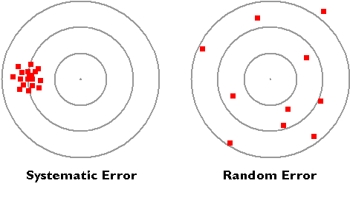# What is Random Errors?

It is very common that repeated measurements of a quantity give values which are slightly different from each other. These errors have no set pattern and occur in a random manner. Hence they are called random errors.

They can be minimized by repeating the measurements many times and taking the arithmetic mean of all the values as the correct reading.

The most common way of expressing an error is percentage error. If the accuracy in measuring a quantity x is Δx, then the percentage error in x is given by [Δx/x * 100 %]